# Deformation Gradient Civil Engineering (CE) Notes | EduRev

## Civil Engineering (CE) : Deformation Gradient Civil Engineering (CE) Notes | EduRev

The document Deformation Gradient Civil Engineering (CE) Notes | EduRev is a part of the Civil Engineering (CE) Course Advanced Solid Mechanics - Notes, Videos, MCQs & PPTs.
All you need of Civil Engineering (CE) at this link: Civil Engineering (CE)

The gradient of motion is generally called the deformation gradient and is denoted by F. Thus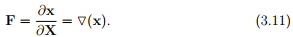Since, χ is a function of both X and t we have used a partial derivative in the definition of the deformation gradient. Also, we haven’t defined it as Grad(x) because for the Grad operator, as defined in chapter 2, the range of the function for which gradient is sought is any vector; not just position vectors. The difference becomes evident in curvilinear coordinate systems like the cylindrical polar coordinates.

Let {Eibe the three Cartesian basis vectors in the reference configuration and {eithe basis vectors in the current configuration. Then, the deformation gradient is written as

F = Fijei ⊗ Ej .                                                     (3.12)

In general, the basis vectors ei and Ej need not be the same. Since the deformation gradient depends on two sets of basis vectors, it is called a twopoint tensor. It is pertinent here to point out that the grad operator as defined in chapter 2 (2.207), is not a two-point tensor either. The matrix components of the deformation gradient in Cartesian coordinate system is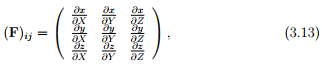where (X, Y, Z) and (x, y, z) are the Cartesian coordinates of a typical material particle, P in the reference and current configuration respectively. Similarly, the matrix components of the deformation gradient in cylindrical polar coordinate system is: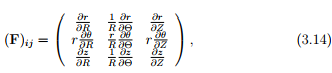where (R, Θ, Z) and (r, θ, z) are the cylindrical polar coordinates of a typical material particle, P in the reference and current configuration respectively. Substituting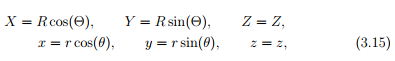in (3.13) we obtain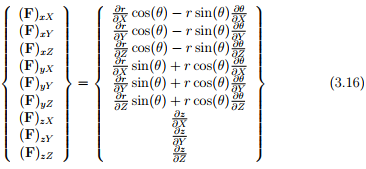where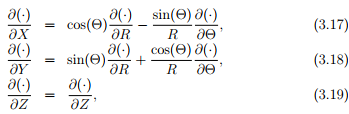from which we obtain (3.14) recognizing that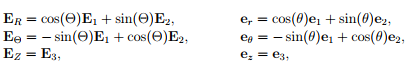where {ER, EΘ, EZ} and {er, eθ, ez} are the cylindrical polar coordinate basis vectors obtained from (2.242) using (3.15). Comparing equations (3.14) with (2.259) we see the difference between the Grad operator and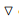operator

Offer running on EduRev: Apply code STAYHOME200 to get INR 200 off on our premium plan EduRev Infinity!

## Advanced Solid Mechanics - Notes, Videos, MCQs & PPTs

42 videos|61 docs

,

,

,

,

,

,

,

,

,

,

,

,

,

,

,

,

,

,

,

,

,

;